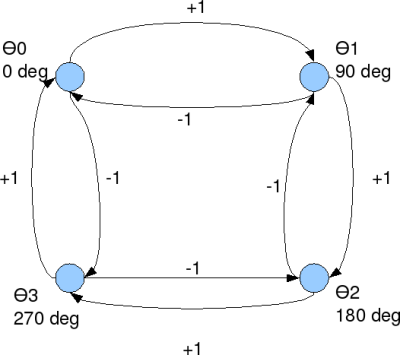- DSP log - http://www.dsplog.com -

Simulating Minimum Shift Keying Transmitter

Posted By Krishna Sankar On January 19, 2008 @ 5:51 am In Modulation | 17 Comments

Minimum shift keying (MSK) is an important concept to learn in digital communications. It is a form of continuous phase frequency shift keying . In minimum phase shift keying, two key concepts are used.

(a) The frequency separation of the sinusoidals used for representing bits 1′s and 0′s are , where is the symbol period.

(b) It is ensured that the resulting waveform is phase continuous.

Motivation of continuous phase

In a previous post (here), we have understood that the minimum frequency separation for two sinusoidals having zero phase difference to be orthogonal is , where is the symbol period. However, it can be observed that at each symbol boundary, there is a phase discontinuity. The presence of phase discontinuities can result in large spectral side lobes outside the desired bandwidth. Hence the need for having a frequency modulated signal which is phase continuous.

Phase growth in MSK

To ensure continuous phase, the phase of the carrier of the MSK signal is
, where

is the symbol period,
corresponds to -1 for bit 0, +1 for bit 1 respectively.

The corresponding carrier signal is
.Figure: Phase transition diagram for MSK (Ref: Fig10.22 in [COMM-PS ]

Simulation Model

Simple Octave/Matlab code for simulating and plotting binary Minimum Shift Keying is kept here .

Next steps

We have observed that bit error probability of classical coherent binary frequency shift keying  is 3dB poorer compared to bit error probability of binary phase shift keying . However, in minimum shift keying, using the knowledge of the phase transitions, we should be able to recover the 3dB loss associated with FSK and get a performance comparable to BPSK. We will hopefully discuss that in a future post.

Reference

URL to article: http://www.dsplog.com/2008/01/19/simulating-minimum-shift-keying-transmitter/

URLs in this post:

 frequency shift keying : http://www.dsplog.com/2007/08/30/bit-error-rate-for-frequency-shift-keying-with-coherent-demodulation/

 here), : http://www.dsplog.com/2007/12/31/minimum-frequency-spacing-for-having-orthogonal-sinusoidals/

 COMM-PS: http://www.amazon.com/Communication-Systems-Engineering-John-Proakis/dp/0130617938